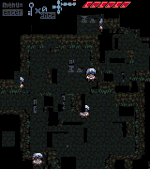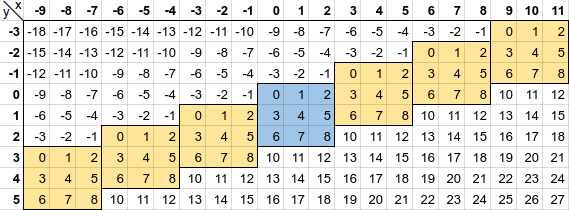## FANDOM

156 PagesPU -1 in the Street showing the out-of-bounds glitch effect

A Parallel Universe (PU for short) is an area found outside a map that appears somewhat like the normal map. While in a PU, you will constantly experience the out-of-bounds glitch effect.

PUs are found west and east of the home universe, not north or south. They contain only BG1 tiles and their physics, not BG2 or FG tiles, and they contain no objects. "PU-1" means the first PU west of the home universe, "PU-2" is the next one after that, etc. "PU+1" is the first PU east of the home universe, and "PU+2" is the next one after that, etc.

## Technical DescriptionEdit

Each map has absolute left, right, top, and bottom edges. Beyond these edges, you get the out-of-bounds glitch effect. The distance between the left and right edges of the map is the absolute width of the map.

The tiles at each location in the map are stored in a 1-dimensional array in row-major order, not a 2-dimensional array. For a 3x3 map, the array indexes would be this (they start at 0):

```012
345
678```

The tile at each location on the screen is calculated from the tile's (x,y) coordinates with this formula:

```tile_offset = y * width + x;
tile_id = tile_data[tile_offset];```

Examples in the 3x3 map above:

• upper left corner: `(0,0) -> tile_offset = 0 * 3 + 0 = 0`
• middle tile: `(1,1) -> tile_offset = 1 * 3 + 1 = 4`
• bottom center tile: `(1,2) -> tile_offset = 2 * 3 + 1 = 7`

If the (x,y) location of a tile is out of bounds, this formula will give some believable numbers anyway sometimes:

• to the right of tile 2: `(3,0) -> tile_offset = 0 * 3 + 3 = 3`
• to the left of tile 3: `(-1,1) -> tile_offset = 1 * 3 + -1 = 2`
• below tile 7: `(1,3) -> tile_offset = 3 * 3 + 1 = 11`
• above tile 1: `(1,-1) -> tile_offset = -1 * 3 + 1 = -2`
• above and right of tile 2: `(3,-1) -> tile_offset = -1 * 3 + 3 = 0`
• below and left of tile 6: `(-1,3) -> tile_offset = 3 * 3 + -1 = 8`

Any tile_offset outside the range of the visible map (such as 11 and -2 above) will be the "default tile", which is usually a transparent (often black) tile with floor physics. The exception is in BLANK and GO where the "default tile" tile has wall physics instead.

You can see from these examples, that you get apparently in-bounds tile offsets while out of bounds to the left and right. These apparently in-bounds tiles comprise the parallel universes.The continuum of a 3x3 map. The Home Universe is in blue, and the PU-3 to PU+3 are in yellow. White cells are the "default tile".

See illustration for how each PU is vertically offset by 1 row from its adjacent universe.

Note that realistically, maps are 30-120 tiles wide, not 3, so the vertical offset is rather subtle when visualized to scale.